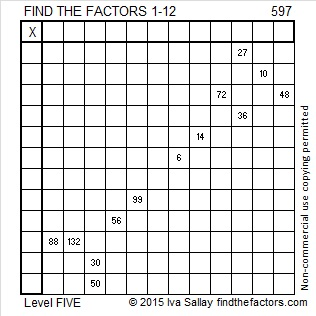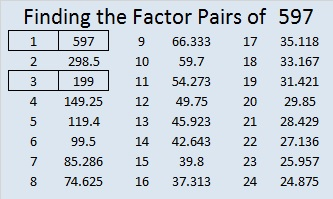# 597 and Level 5

597 is made from three consecutive odd numbers so it is divisible by 3.

Because it is divisible by 3, it is the sum of three consecutive numbers. It is also the sum of three consecutive odd numbers:

• 198 + 199 + 200 = 597
• 197 + 199 + 201 = 597

Why was 199 the middle number in both of those sums?

OEIS.org informs us that even though (597!!! + 1) generates an error message on our calculators, it is indeed a very large prime number. Whoever discovered that fact must have been extremely excited.Print the puzzles or type the solution on this excel file: 12 Factors 2015-08-24

—————————————————————————————————

• 597 is a composite number.
• Prime factorization: 597 = 3 x 199
• The exponents in the prime factorization are 1 and 1. Adding one to each and multiplying we get (1 + 1)(1 + 1) = 2 x 2 = 4. Therefore 597 has exactly 4 factors.
• Factors of 597: 1, 3, 199, 597
• Factor pairs: 597 = 1 x 597 or 3 x 199
• 597 has no square factors that allow its square root to be simplified. √597 ≈ 24.43358—————————————————————————————————This site uses Akismet to reduce spam. Learn how your comment data is processed.